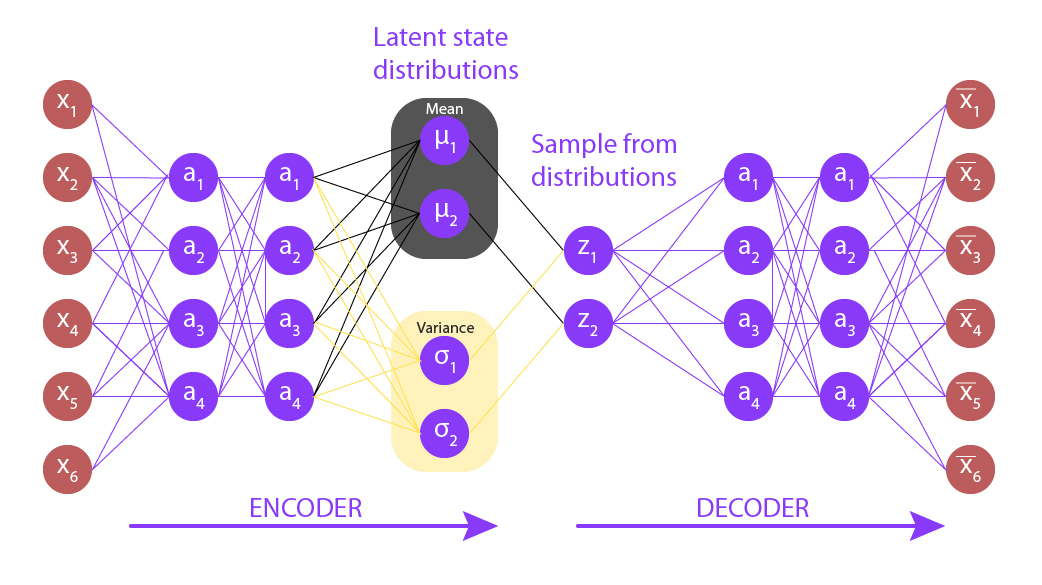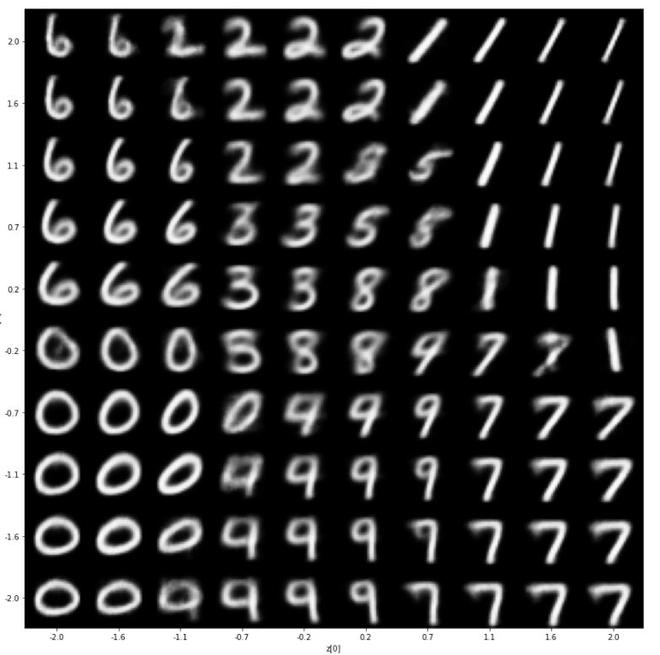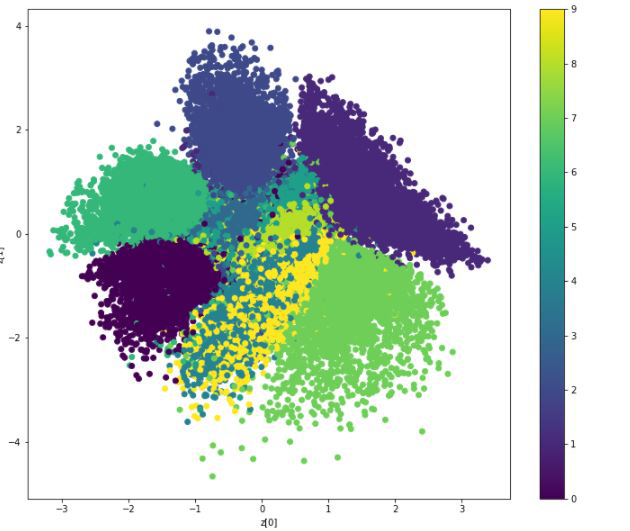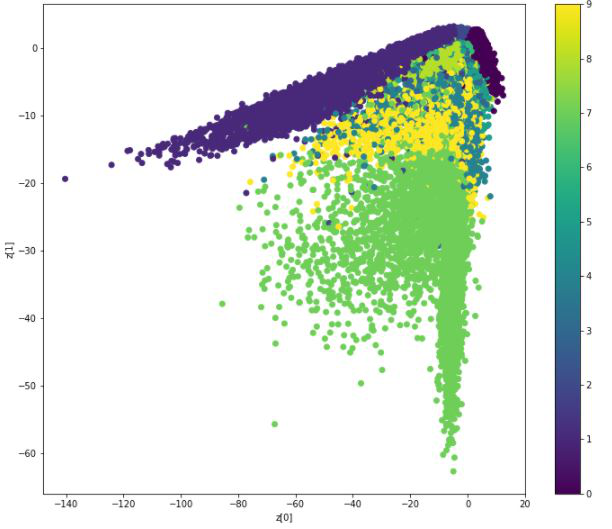Related Articles

# Role of KL-divergence in Variational Autoencoders

• Last Updated : 16 Sep, 2021

### Variational Autoencoders

Variational autoencoder was proposed in 2013 by Knigma and Welling at Google and Qualcomm. A variational autoencoder (VAE) provides a probabilistic manner for describing an observation in latent space. Thus, rather than building an encoder that outputs a single value to describe each latent state attribute, we’ll formulate our encoder to describe a probability distribution for each latent attribute.

#### Architecture:

Autoencoders basically contains two parts:

• The first one is an encoder which is similar to the convolution neural network except for the last layer. The aim of the encoder is to learn efficient data encoding from the dataset and pass it into a bottleneck architecture.
• The other part of the autoencoder is a decoder that uses latent space in the bottleneck layer to regenerate the images similar to the dataset. These results backpropagate from the neural network in the form of the loss function.

Variational autoencoder is different from autoencoder in a way such that it provides a statistical manner for describing the samples of the dataset in latent space. Therefore, in the variational autoencoder, the encoder outputs a probability distribution in the bottleneck layer instead of a single output value.### Explanation:

The Variational Autoencoder latent space is continuous. It provides random sampling and interpolation. Instead of outputting a vector of size n, the encoder outputs two vectors:

• Vector ????  of means (vector size n)
• Vector ???? of standard deviations (vector size n)

Output is an approximate posterior distribution q(z|x). Sample from this distribution to get z. let’s look at more details into Sampling:

• Let’s take some values of mean and standard deviation,
•• The intermediate distribution that is generated from that:
•• Now, let’s generate the sampled vector from this:
•• While the mean and standard deviation are the same for one input the result may be different due to sampling.
• Eventually, our goal is to make the encoder learn to generate differently???? For different classes, clustering them and generating encoding such they don’t vary much. To this we use KL-divergence.

### KL-divergence:

KL divergence stands for Kullback Leibler Divergence, it is a measure of divergence between two distributions. Our goal is to Minimize KL divergence and optimize ???? and ???? of one distribution to resemble the required distribution.Of

For multiple distribution the KL-divergence can be calculated as the following formula:where X_j \sim N(\mu_j, \sigma_j^{2})  is the standard normal distribution.

### VAE Loss:

Suppose we have a distribution z and we want to generate the observation x from it.  In other words, we want to calculateWe can do it by following way:But, the calculation of p(x) can be quite difficultThis usually makes it an intractable distribution. Hence, we need to approximate p(z|x) to q(z|x) to make it a tractable distribution. To better approximate p(z|x) to q(z|x), we will minimize the KL-divergence loss which calculates how similar two distributions are:By simplifying, the above minimization problem is equivalent to the following maximization problem :The first term represents the reconstruction likelihood and the other term ensures that our learned distribution q is similar to the true prior distribution p.

Thus our total loss consists of two terms, one is reconstruction error and the other is KL-divergence loss:### Implementation:

In this implementation, we will be using the MNIST dataset, this dataset is already available in keras.datasets API, so we don’t need to add or upload manually.

• First, we need to import the necessary packages to our python environment. we will be using Keras package with TensorFlow as a backend.

## python3

 # codeimport numpy as npimport tensorflow as tffrom tensorflow import kerasfrom tensorflow.keras import Input, Modelfrom tensorflow.keras.layers import Layer, Conv2D, Flatten, Dense, Reshape, Conv2DTransposeimport matplotlib.pyplot as plt
• For variational autoencoders, we need to define the architecture of two parts encoder and decoder but first, we will define the bottleneck layer of architecture, the sampling layer.

## python3

 # this sampling layer is the bottleneck layer of variational autoencoder,# it uses the output from two dense layers z_mean and z_log_var as input, # convert them into normal distribution and pass them to the decoder layerclass Sampling(Layer):      def call(self, inputs):        z_mean, z_log_var = inputs        batch = tf.shape(z_mean)        dim = tf.shape(z_mean)        epsilon = tf.keras.backend.random_normal(shape =(batch, dim))        return z_mean + tf.exp(0.5 * z_log_var) * epsilon
• Now, we define the architecture of the encoder part of our autoencoder, this part takes images as input and encodes their representation in the Sampling layer.

## python3

 # Define Encoder Modellatent_dim = 2  encoder_inputs = Input(shape =(28, 28, 1))x = Conv2D(32, 3, activation ="relu", strides = 2, padding ="same")(encoder_inputs)x = Conv2D(64, 3, activation ="relu", strides = 2, padding ="same")(x)x = Flatten()(x)x = Dense(16, activation ="relu")(x)z_mean = Dense(latent_dim, name ="z_mean")(x)z_log_var = Dense(latent_dim, name ="z_log_var")(x)z = Sampling()([z_mean, z_log_var])encoder = Model(encoder_inputs, [z_mean, z_log_var, z], name ="encoder")encoder.summary()
Model: "encoder"
__________________________________________________________________________________________________
Layer (type)                    Output Shape         Param #     Connected to
==================================================================================================
input_3 (InputLayer)            [(None, 28, 28, 1)]  0
__________________________________________________________________________________________________
conv2d_2 (Conv2D)               (None, 14, 14, 32)   320         input_3
__________________________________________________________________________________________________
conv2d_3 (Conv2D)               (None, 7, 7, 64)     18496       conv2d_2
__________________________________________________________________________________________________
flatten_1 (Flatten)             (None, 3136)         0           conv2d_3
__________________________________________________________________________________________________
dense_2 (Dense)                 (None, 16)           50192       flatten_1
__________________________________________________________________________________________________
z_mean (Dense)                  (None, 2)            34          dense_2
__________________________________________________________________________________________________
z_log_var (Dense)               (None, 2)            34          dense_2
__________________________________________________________________________________________________
sampling_1 (Sampling)           (None, 2)            0           z_mean
z_log_var
==================================================================================================
Total params: 69, 076
Trainable params: 69, 076
Non-trainable params: 0
__________________________________________________________________________________________________
• Now, we define the architecture of decoder part of our autoencoder, this part takes the output of the sampling layer as input and output an image of size (28, 28, 1) .

## python3

 # Define Decoder Architecturelatent_inputs = keras.Input(shape =(latent_dim, ))x = Dense(7 * 7 * 64, activation ="relu")(latent_inputs)x = Reshape((7, 7, 64))(x)x = Conv2DTranspose(64, 3, activation ="relu", strides = 2, padding ="same")(x)x = Conv2DTranspose(32, 3, activation ="relu", strides = 2, padding ="same")(x)decoder_outputs = Conv2DTranspose(1, 3, activation ="sigmoid", padding ="same")(x)decoder = Model(latent_inputs, decoder_outputs, name ="decoder")decoder.summary()
Model: "decoder"
_________________________________________________________________
Layer (type)                 Output Shape              Param #
=================================================================
input_4 (InputLayer)         [(None, 2)]               0
_________________________________________________________________
dense_3 (Dense)              (None, 3136)              9408
_________________________________________________________________
reshape_1 (Reshape)          (None, 7, 7, 64)          0
_________________________________________________________________
conv2d_transpose_3 (Conv2DTr (None, 14, 14, 64)        36928
_________________________________________________________________
conv2d_transpose_4 (Conv2DTr (None, 28, 28, 32)        18464
_________________________________________________________________
conv2d_transpose_5 (Conv2DTr (None, 28, 28, 1)         289
=================================================================
Total params: 65, 089
Trainable params: 65, 089
Non-trainable params: 0
_________________________________________________________________
• In this step, we combine the model and define the training procedure with loss functions.

## python3

 # this class takes encoder and decoder models and# define the complete variational autoencoder architectureclass VAE(keras.Model):    def __init__(self, encoder, decoder, **kwargs):        super(VAE, self).__init__(**kwargs)        self.encoder = encoder        self.decoder = decoder      def train_step(self, data):        if isinstance(data, tuple):            data = data        with tf.GradientTape() as tape:            z_mean, z_log_var, z = encoder(data)            reconstruction = decoder(z)            reconstruction_loss = tf.reduce_mean(                keras.losses.binary_crossentropy(data, reconstruction)            )            reconstruction_loss *= 28 * 28            kl_loss = 1 + z_log_var - tf.square(z_mean) - tf.exp(z_log_var)            kl_loss = tf.reduce_mean(kl_loss)            kl_loss *= -0.5            # beta =10            total_loss = reconstruction_loss + 10 * kl_loss        grads = tape.gradient(total_loss, self.trainable_weights)        self.optimizer.apply_gradients(zip(grads, self.trainable_weights))        return {            "loss": total_loss,            "reconstruction_loss": reconstruction_loss,            "kl_loss": kl_loss,        }
• Now it’s the right time to train our variational autoencoder model, we will train it for 100 epochs.  But first we need to import the MNIST dataset.

## python3

 # load fashion mnist dataset  from  keras.dataset API(x_train, _), (x_test, _) = keras.datasets.fashion_mnist.load_data()fmnist_images = np.concatenate([x_train, x_test], axis = 0)# expand dimension to add  a color map dimensionfmnist_images = np.expand_dims(fmnist_images, -1).astype("float32") / 255  # compile and train the modelvae = VAE(encoder, decoder)vae.compile(optimizer ='rmsprop')vae.fit(fmnist_images, epochs = 100, batch_size = 128)
• In this step, we display training results, we will be displaying these results according to their values in latent space vectors.

## python3

 def plot_latent(encoder, decoder):    # display a n * n 2D manifold of imagess    n = 10    img_dim = 28    scale = 2.0    figsize = 15    figure = np.zeros((img_dim * n, img_dim * n))    # linearly spaced coordinates corresponding to the 2D plot    # of images classes in the latent space    grid_x = np.linspace(-scale, scale, n)    grid_y = np.linspace(-scale, scale, n)[::-1]      for i, yi in enumerate(grid_y):        for j, xi in enumerate(grid_x):            z_sample = np.array([[xi, yi]])            x_decoded = decoder.predict(z_sample)            images = x_decoded.reshape(img_dim, img_dim)            figure[                i * img_dim : (i + 1) * img_dim,                j * img_dim : (j + 1) * img_dim,            ] = images      plt.figure(figsize =(figsize, figsize))    start_range = img_dim // 2    end_range = n * img_dim + start_range + 1    pixel_range = np.arange(start_range, end_range, img_dim)    sample_range_x = np.round(grid_x, 1)    sample_range_y = np.round(grid_y, 1)    plt.xticks(pixel_range, sample_range_x)    plt.yticks(pixel_range, sample_range_y)    plt.xlabel("z")    plt.ylabel("z")    plt.imshow(figure, cmap ="Greys_r")    plt.show()    plot_latent(encoder, decoder)Output from Encoder

• To get a more clear view of our representational latent vectors values, we will be plotting the scatter plot of training data on the basis of their values of corresponding latent dimensions generated from the encoder

## python3

 def plot_label_clusters(encoder, decoder, data, test_lab):    z_mean, _, _ = encoder.predict(data)    plt.figure(figsize =(12, 10))    sc = plt.scatter(z_mean[:, 0], z_mean[:, 1], c = test_lab)    cbar = plt.colorbar(sc, ticks = range(10))    cbar.ax.set_yticklabels([i for i in range(10)])    plt.xlabel("z")    plt.ylabel("z")    plt.show()  (x_train, y_train), _ = keras.datasets.mnist.load_data()x_train = np.expand_dims(x_train, -1).astype("float32") / 255plot_label_clusters(encoder, decoder, x_train, y_train)Distribution for Beta = 10

• To compare the difference, I also train the above autoencoder for \beta =0 i.e we remove the Kl-divergence loss, and it generated the following distribution:Distribution for Beta = 0

Here, we can see that the distribution is not separable and quite skewed for different values, that’s why we use KL-divergence loss in the above variational autoencoder.

References:

Attention reader! Don’t stop learning now. Get hold of all the important Machine Learning Concepts with the Machine Learning Foundation Course at a student-friendly price and become industry ready.

My Personal Notes arrow_drop_up